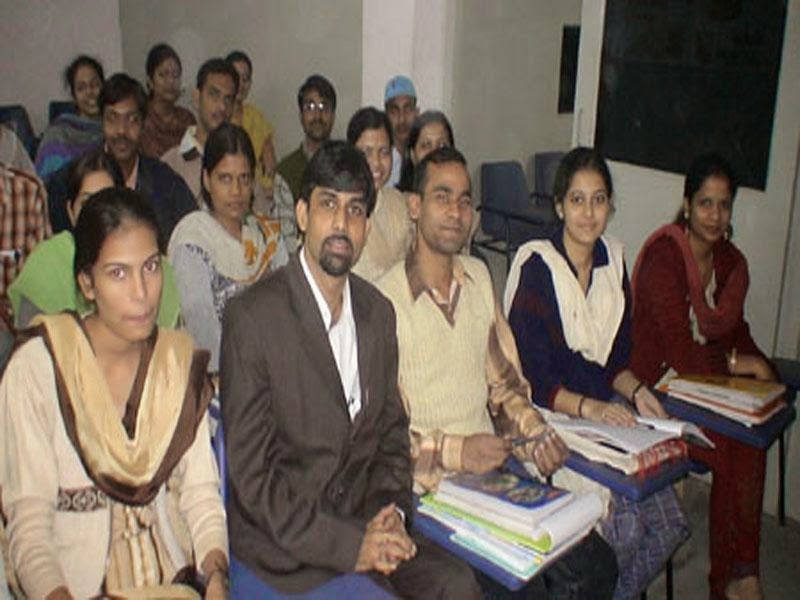# Syllabus Dips Academy-Csir mathematics Part (3)IISc
polynomials, diagonalizability, Jordan canonical form. Abstract Algebra : Groups: subgroups, Lagrange’s theorem, normal subgroup, quotient group, homomorphism, permutation groups, Cayley’s theorem, Sylow theorems, Rings, Ideals Fields. Ordinary Differential Equations : First order ODEs and their solutions, singular solutions, experience and uniqueness of initial value problems for first order ODE. Gewneral theory of homogeneous and homomor-geneous linear differential
equations. Variation of parameters. Types of singular points in the phase plane of an autonomous system of two equations. Partial Differential Equations: Elements of first order PDE. Second order linear PDE: Classification, wave Laplace and Heat equations. Basic properties and important solutions of classical initial and boundary value problems. Elements of Numerical Analysis: Interpolation : Lagrange and Newton’s forms, error in interpolation. Solution of nonlinear equations by iteration, various iterative methods including Newton. Raphson method, fixed point iteration. Convergence, integration: trapezoidal rule, Simpson’s rule, Gaussian rule, expressions for the error terms. Solution of ordinary differential equations: simple difference equations, series method, Euler’s method, Runge Kutta methods, predictor- corrector methods, error estimates.

MCA Entrance Exam
Algebra:
Fundamental operations in Algebra, Expansions, Factorization, simultaneous linear and quadratic equations, indices, logarithms, arithmetic, geometric and harmonic progressions, binomial theorem, permutations and combinations, surds, determinants, matrices and application to solution of simultaneous linear equations, Set Theory, Group Theory. Coordinate Geometry: Rectangular Cartesian coordinates, equations of a line, midpoint, intersections etc., equations of a circle, distance formulae, pair of straight lines, parabola, ellipse and hyperbola, simple geometric transformations such as translation, rotation, scaling. Calculus: Limit of functions, continuous functions, differentiation of functions tangents and normals, simple examples of maxima and minima, Integration of function by parts, by substitution and by partial fraction, definite integrals, and applications of Definite Integrals to areas. Differential Equations: Differential equations of first order and their solutions, linear differential
equations with constant coefficients, homogenous
linear differential equations.
Vector: Position Vector,
vectors, scalar and vector
products and their
applications to simple
geometrical problems and
mechanics.

Trigonometry: Simple identities, trigonometric equations, properties of triangles, solution of triangles, height and distance, inverse function, Inverse Trigonometric functions, General solutions of trigonometric equations, Complex numbers. Real Analysis: Sequence of real numbers, Convergent Sequences, Cauchy’s Sequences, Monotonic Sequences, Infinite series and their different tests of convergence, The only way to gain respect is, firstly to give it DIPS Academy /14

JNU
Absolute convergence, Uniform convergence, properties of continuous functions, Rolle’s theorem, Mean value theorem, Taylor’s and Maclaurian’s series, Maxima and Minima, Indeterminate forms.
Statistics & Linear Programming: Frequency distribution and measure of dispersion, skewness and Kurtosis, Permutations and Combinations, Probability, Random variables and distribution function, Mathematical expectation and generating function, Binomial, Poisson normal distribution curve fitting and principle of least squares, Correlation and Regression, Sampling and large sample tests, Test of significance base on t, x2 and f distribution, Formulation of simple linear programming problems, basic concepts of graphical and simple methods.
Analytical Ability and Logical Reasoning
The questions in this section will cover logical reasoning, quantitative reasoning.
ComputerAwareness

Computer Basics: Organization of a Computer, Central Processing Unit (CPU), Structure of instructions in CPU, input/output devices, computer memory, memory organization, back- up devices.
Data Representation: Representation of characters, integers and fractions, binary and hexadecimal representations, Binary Arithmetic: Addition, subtraction, division, multiplication, single arithmetic and two’s complement arithmetic, floating point representation of numbers, normalized floating point representation, Boolean algebra, truth tables, Venn diagrams.
Elements of Data Structures Computer Organization C Language

Resource:- net-mathematics

This post first appeared on Dips Academy: NET/JRF, IIT-JAM, IAS And GATE Coach, please read the originial post: here

# Share the post

Syllabus Dips Academy-Csir mathematics Part (3)

×